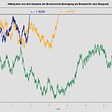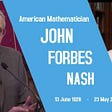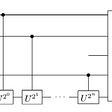# RL — Actor-Critic using Kronecker-Factored Trust Region (ACKTR) Explained

In a previous article, we explain how Natural Policy Gradient allows the Policy Gradient methods to converge better by not making bad moves that destroy training performance. However, Natural Policy Gradient is a second-order optimization method which is much slower than a first-order optimization. In this article, we will introduce ACKTR which will reduce the complexity closer to a first-order optimization like the Gradient Descent.

In the figure below, it shows ACKTR outperforms some very popular RL methods like A2C and TRPO.

# Trust Region Policy Optimization TRPO

Recall that Natural Policy Gradient is:

However, computing F and its inverse is expensive.

TRPO addresses the later problem by using the conjugate gradient to approximate:

We transform the problem above to an optimization problem in the quadratic equation form. Conjugate gradient is similar to the gradient descent but much more efficient for a quadratic equation. It locates the optimum in at most N iterations where N is the number of parameters in the policy model θ. This sounds complicated but it is computational less complex than finding the inverse of F.

So how can we transform the problem into an optimization problem? We transform the linear equation to

and use the conjugate gradient to optimize the corresponding quadratic equation as:

To approximate x, we optimize:

TRPO shortcomings

For each model parameter update, we still need to compute F. This hurts scalability:

• Computing F every time for the current policy model is expensive, and
• It requires a large batch of rollouts to approximate F.

We use an alternative equation below to compute F. But to be accurate, it needs to sample a lot of data.

Because of this scalability issue, TRPO is not practical for large deep networks. Empirically, it does not perform well on tasks requiring deep convolutional or RNN networks. The use of the conjugate gradient also complicates the implementation. We need a better and scalable way to compute F. That is what ACKTR good at.

# Actor Critic using Kronecker-Factored Trust Region (ACKTR)

ACKTRK is:

• A Policy Gradient method with the trust region optimization,
• An actor-critic architecture similar to A2C: one deep network to estimate the policy and another network to estimate the advantage function,
• Apply the Kronecker-factored approximation to optimize both actor and critic (will be explained next),
• Keep a running average of the curvature information.

ACKTR speeds up the optimization by reducing the complexity of calculating the inverse of the F (FIM) using the Kronecker-factored approximation. Comparing with the first-order optimization like the stochastic gradient descent, ACKTRK adds only about 10%-25% more computation cost.

# Kronecker-factored Approximate Curvature (K-FAC)

Second-order optimization is more accurate but the increase of complexity usually cannot justify for its return compared with the first-order optimization even in RL. Compute a Hessian matrix is in the order of

This is not feasible for larger deep network models. K-FAC approximates the inverse of FIM and computes the parameter updates one network layer at a time. Each calculation only involves the nodes in a layer instead of all the nodes in the whole model. Therefore, the computational complexity drops significantly.

K-FAC calculates the derivative of L relative to model parameters w in a deep network layer, instead of the whole model. After applying the chain rule, the partial derivative of L relative to the parameters W in a layer becomes:

As shown before, F can be calculated as:

The corresponding calculation for each layer becomes:

Apply the partial derivates shown before, the calculation is simplified to:

with the outer product of two vectors defined as:

Applying

The updates to W for each layer become:

So to compute the parameter changes for each layer, we find the inverse of A and S below:

We update the network layer-by-layer. Each calculation involves nodes in each layer only. Consider the number of nodes in each layer is in thousand or hundred ranges, rather than millions for the whole network. This is much manageable and K-FAC significantly reduces the computational complexity. Even the method in the paper is for the dense network, K-FAC approximation for convolutional networks has also been developed by other researchers.

In TRPO, the variance in computing F is high. To improve accuracy, many samples are collected in calculating F.

K-FAC maintains running averages for the expectations A and S in the approximation. This reduces variance and therefore K-FAC requires far fewer samples. Empirical results prove that ACKTR is more sample efficient.

# Critic

ACKTR uses a critic to estimate the advantage function. To train the model, we compare the model predictions with the target values (r(x) = estimated(x) — target(x)) and solve the problem as a least-square problem (a.k.a. mean square error MSE). One common approach with a second-order algorithm is the Gauss-Newton. If we compare the actor and the critic side-by-side, the equations in updating the model parameters are in similar form.

The FIM F is equivalent to the Gauss-Newton matrix in the critic (the underlined red line above). Hence, we can apply similar K-FAC approximation method to the critic and update each layer one-by-one to reduce the computational complexity.

The following is the performance improvement in applying the Gauss-Newton norm (a second-order optimization) rather than the usual Euclidean norm (first-order) to the critic in ACKTR.

# Result

ACKTR

• uses trust-region optimization to improve the convergence.
• approximates the parameter updates with K-FAC for both actor and critic. It reduces the computational complexity closer to first-order optimizers (like the common stochastic gradient descent).
• maintains running averages to reduce variance in the K-FAC calculation. This allows K-FAC to use less sample than TRPO to approximate the inverse of F accurately.

ACKTR scales better with larger deep network models. It solves tasks in continuous control space with raw pixel observation which TRPO cannot handle.

Sample efficiency

ACKTR scores higher rewards (the rewards column) and achieves the human level performance in fewer training episodes (the episode column).

Below shows the number of time steps executed per second (the higher the better). ACKTR achieves speed performance within 25% of A2C which uses a first-order optimization.

Limitations

ACKTR is less straightforward for RNN or other shared parameters networks.

--

--

--

## More from Jonathan Hui

Deep Learning

Love podcasts or audiobooks? Learn on the go with our new app.

## Download In >PDF GRE Math Strategies: Effective Strategies & Practice from 99th Percentile…## Geodesic Regression## Practice Algorithms with Math Puzzles Part 2## What’s Atomic Packing Factor?## Continuous Time Martingales for Quantitative Finance## John Nash : A Mathematical Genius## Quantum Phase Estimation## Leading Digits and Benford’s LawDeep Learning

## Caveats & Limitations on AI Fairness Approaches## Everything you need to know about Reinforcement Learning in <80minutes## Deep Q-Networks